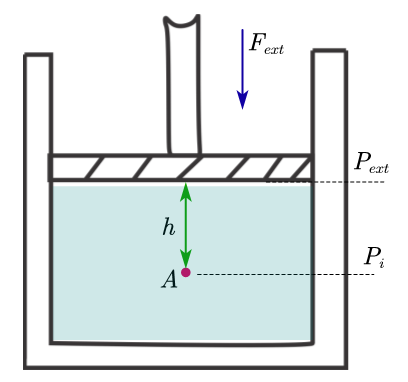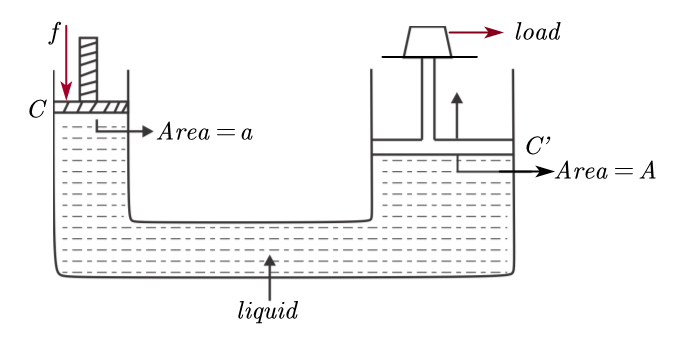# Pascals Law

## What is Pascal's Law

-This law constitutes one of the basic principles of hydrostatics.
-statement of Pascal's law is,
"If the pressure in an incompressible liquid is changed at a particular point, the change is transmitted without diminishing in magnitude to all other parts of the fluid and to the walls of its container”
-To demonstrate the Pascal's law consider a flask fluid with incompressible fluid and open end of flask is flitted with moveable piston shown below in the figure.Figure:- proof of Pascal’s law
-If we apply external force on the piston then pressure on the piston and on the liquid would be $\frac {F_{ent}}{A}$ where A is the cross-section
-Initially pressure $P_i$ at any point A on the liquid is
$P_i= \frac {F_{ent}}{A} + \rho gh$ $=P_{ent} + \rho gh$ - Now if we increase the force exerted by an amount $\Delta F_{ent}$ on piston,then the pressure is also increased by an amount $\Delta P_{ent}$. Now final pressure $P_f$ at point A is
$P_f= P_{ent} + \Delta P_{ent} + \rho gh$
$P_f=P_i + \Delta P_{ent}$
$\Delta P = P_f - P_i= \Delta P_{ent}$
Here we can see that this change in pressure is independent of height h, hence it must hold for all points in the fluid. This is what the Pascal’s law indicates.

## Experimental verification of Pascal's law

figure
• Take a vessel having three side takes A , B and C which are fitted with friction less and water tight pistons.
• As shown in the figure, the cross-sectional areas of the pistons A , B and C are a, 2a and a/2 resp.
• Vessel is now filled with water and an additional force f is applied on position A.
• To keep pistons B and C in their positions, forces equal to 2f and f/2 resp. have to be applied on them. This shows that the pressure is transmitted equally in all directions because
$P=\frac{F}{A}= \frac {2F}{2A}= \frac {F/2}{A/2}$
• This experiment shows that pressure is transmitted undiminished in all directions.

## Hydraulic Lift

• Hydraulic lift is an important application of Pascal’s law.
• Hydraulic lift is used to support or lift heavy objects. It is a force multiplier.
• Hydraulic lifts are used to lift vehicles at a service station.
Principle
when a small force is applied over a piston of small cross-sectional area, then due to transmission of pressure equally in all directions, a large force appear over a piston of large cross-sectional area, which is then utilized to support and lift heavy weights.
Construction
• A hydraulic lift consists of two cylinders C and C' connected to each other with a pipe.
• The two cylinders are of different areas of cross-section and are fitted with frictionless and water tight pistons. As shown below in the figure.• Now a force f is applied on a smaller piston of cross-sectional area 'a'
In this case pressure exerted over the liquid would be
$P=\frac {f}{a}$
According to Pascal’s law, the same amount of pressure is transmitted to the larger piston of larger cross-sectional area A.
• So, the force transmitted to the larger piston is given by
$F=PA$
$F= \frac {f}{a} A$
As $A>a$
therefore $F>f$
• Since A is greater than a, force f on the larger piston will also be much larger than the force f applied on the smaller piston.
• So if we are able to make ratio of areas of pistons (A/a) large, we can lift very heavy loads (like cars, trucks etc.) by applying small amount of force

## Hydraulic Breaks

• hydraulic breaks used in automobiles are based on Pascal's law of transmission of pressure in a liquid.
• Figure below shows a diagram of a part of hydraulic system.
Figure Hydraulic Break
• As shown in the figure one end of the tube T containing break oil is connected to master cylinder with a piston P.
• The other end of the tube is connected to a wheel cylinder having two pistons $P_1$ and $P_2$
• These two Pistons are then connected to the brea shoes $S_1$ and $S_2$ respectively.
• The Piston P of the master cylinder is connected to a pedal through a lever system.
Working
• When the driver of the vehicle puts pressure on the brake paddle the lever system moves a piston. P into the master cylinder containing the break fluid as shown in the figure given above.
• The break fluid from the master cylinder is led through strong pipes to cylinders provided with pistons $P_1$ and $P_2$ of larger cross-sectional area.
• The pressure is transmitted in accordance with Pascal's law.
• Here the small force applied over the break paddle is transmitted by the break fluid as a large force to the piston of for each break.
• The pistons $P_1$ and $P_2$ pushes the break shoes away from each other.
• These break shoes press against the inner rim of the wheel and retard the motion of the wheel.
• In order to apply equal pressure to all the wheels, the master cylinder is connected to all the wheels of the vehicle through tubes.
• when the paddle is released, a spring system brings the break shoe back to their normal positions and the break fluid returns to the master cylinder.